## Example Questions

← Previous 1 3 4

### Example Question #1 : Integers

Which of the following could represent the sum of 3 consecutive odd integers, given that d is one of the three?

3d – 3

3d + 4

3d – 6

3d – 9

3d + 3

3d – 6

Explanation:

If the largest of the three consecutive odd integers is d, then the three numbers are (in descending order):

dd – 2, d – 4

This is true because consecutive odd integers always differ by two. Adding the three expressions together, we see that the sum is 3d – 6.

### Example Question #1 : Even / Odd Numbers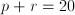, where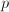andare distinct positive integers.  Which of the following could be values ofand?

–10 and 30

4 and 5

5 and 15

10 and 10

0 and 20

5 and 15

Explanation:

Sinceandmust be positive, eliminate choices with negative numbers or zero. Since they must be distinct (different), eliminate choices where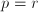.  This leaves 4 and 5 (which is the only choice that does not add to 20), and the correct answer, 5 and 15.

### Example Question #1 : Integers

The sum of three consecutive odd integers is 93. What is the largest of the integers?Explanation:

Consecutive odd integers differ by 2. If the smallest integer is x, then

x + (x + 2) + (x + 4) = 93

3x + 6 = 93

3x = 87

x = 29

The three numbers are 29, 31, and 33, the largest of which is 33.

### Example Question #1 : Integers

Solve: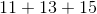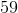Explanation: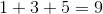Since there is no tens digit to carry over, proceed to add the tens digits: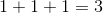The answer is.

### Example Question #2 : Even / Odd Numbers

At a certain high school, everyone must take either Latin or Greek. There aremore students taking Latin than there are students taking Greek. If there are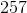students taking Greek, how many total students are there?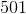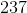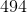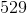Explanation:

If there arestudents taking Greek, then there are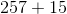or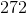students taking Latin. However, the question asks how many total students there are in the school, so you must add these two values together to get: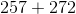ortotal students.

### Example Question #1 : Even / Odd Numbers

Add: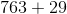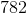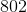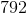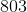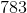Explanation: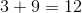Since the there is a tens digit, use that as the carryover to the next term.

Add the tens digit including the carryover.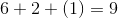The hundreds digit is 7.

Combine the ones digit of each calculation in order.

The answer is:### Example Question #2 : Integers

Add: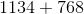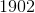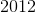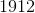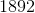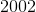Explanation: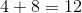Carry over the one from the tens digit to the next number.

Add the tens digit with the carry over.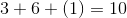Carry over the one from the tens digit to the hundreds digit.

Add the hundreds digit with the carry over.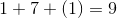The thousands digit has no carry over.  The second number has no thousands digit.  This means that the thousands is one.  Combine all the ones digits from each of the previous calculations.

The correct answer is:### Example Question #1 : Integers

When 8 integers are multiplied their product is negative, then at most how many of the integers can be negative?

5

4

7

1

8

7

Explanation:

When one multiplies two negative numbers (or any even multiple) the result is a positive number. However, when one multiplies three negative numbers (or any odd multiple) the product is negative. If the result of multiplying 8 negatives is odd, the largest number of negative integers will be the largest odd number, in this case 7.

### Example Question #5 : Even / Odd Numbers

If n and m are both positive even integers, which of the following must be odd?

I. (n + 1)(m + 1)

II. nm + 1

III. nm + m

I, II, and III

I and II only

II only

I only

II and III only

I and II only

Explanation:

Let us analyze I, II, and III one at a time.

Because n and m are both even, if we increase either by 1, the result will be an odd number. Thus, n + 1 and m + 1 are both odd. When two odd numbers are multiplied together, the result is always an odd number. Thus (n + 1)(m + 1) must be an odd number.

Because n and m are even, when we multiply two even numbers together, we always get an even number. Thus nm is even. However, when we then add one to an even number, the result will be an odd number. Thus, nm + 1 is odd.

We just established that nm is even. If we subtract an even number from an even number, the result is always even. Thus, nm – m is an even number.

Only choice I and II will always produce odd numbers.

The answer is I and II only.

### Example Question #41 : Integers

odd * odd * odd =

even * even * even

odd * odd

even * even

odd * odd * even

even * odd

odd * odd

Explanation:

The even/odd number properties are good to know. If you forget them, however, it's easy to check with an example.

Odd * odd = odd. If you didn't remember that, a check such as 1 * 3 = 3 will give you the same answer. So if odd * odd = odd, (odd * odd) * odd = odd * odd = odd, just as 3 * 3 * 3 = 27, which is odd. This means we are looking for an answer choice that also produces an odd number. Let's go through them.

even * even = even (2 * 2 = 4)

even * odd = even (2 * 3 = 6)

odd * odd = odd (1 * 3 = 3) This is the correct answer! But just to double check, let's go through the last two.

even * even * even = even * even = even (2 * 2 * 2 = 8)

odd * odd * even = odd * even = even (1 * 3 * 2 = 6)

← Previous 1 3 4

### All SAT Math Resources